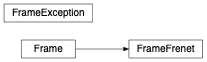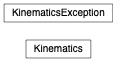# Zgoubidoo’s utility modules¶

## Affine geometry using the frame module¶

Module for handling of affine geometry transformations (rotations and translations).

This module provides support for affine geometry transformations, mainly through the Frame class. A typical use case, the one that triggered the development of this module for ::py:module Zgoubidoo, is the problem of placing a sequence with each object being placed with respect to the one preceeding it, with each object being potentially translate or rotated. In such a case, the ::py:module Frame module allows to define a reference frame for each object being placed, with the reference frame of the newly created object using the reference frame of the previous object as a reference frame for its own positioning. The translations and rotations of the object are thus trivially expressed in its own reference frame. The ::py:module Frame module then allows to query the coordinates and rotation information of the object with respect to any other reference frame in the chain of transformations. In particular, the coordinates of the origin of a frame along with its orientation in space, can be obtained with respect to a global (absolute) reference frame.

Example

example TODO

### Classes¶

 Frame([parent, reference]) A Frame object represents a reference frame for affine geometry transformations (rotations and translations). Exception raised for errors in the Frame module. FrameFrenet([parent, reference]) Initialize a Frame with respect to a parent frame.

### Class Inheritance Diagram¶## Accelerator physics and relativity calculations using the physics module¶

Zgoubidoo relativistic physics module.

This module provides a collection of functions and classes to deal with relativistic physics computations. This mainly concerns conversions between kinematic quantities. Full support for units (via pint) is provided. Additionnally, a helper class (Kinematics) provides automatic construction and conversion of kinematics quantities.

Examples

>>> 1 + 1
TODO


### Functions¶

 beta_to_brho(beta[, particle]) Converts relativistic beta to magnetic rigidity. beta_to_ekin(beta[, particle]) Converts relativistic beta to kinetic energy. beta_to_etot(beta[, particle]) Converts relativistic beta to total energy. beta_to_gamma(beta[, particle]) Converts relativistic beta to relativistic gamma. beta_to_momentum(beta[, particle]) Converts relativistic beta to momentum. beta_to_pv(beta[, particle]) Converts relativistic beta to relativistic pv. beta_to_range(beta[, particle]) Converts relativistic beta to range. brho_to_beta(brho[, particle]) Converts magnetic rigidity (brho) to relativistic beta. brho_to_ekin(brho[, particle]) Converts magnetic rigidity (brho) to kinetic energy. brho_to_etot(brho[, particle]) Converts magnetic rigidity (brho) to total energy. brho_to_gamma(brho[, particle]) Converts magnetic rigidity (brho) to relativistic gamma. brho_to_momentum(brho[, particle]) Converts magnetic rigidity (brho) to momentum. brho_to_pv(brho[, particle]) Converts magnetic rigidity (brho) to relativistic pv. brho_to_range(brho[, particle]) Converts magnetic rigidity (brho) to range. ekin_to_beta(e[, particle]) Converts the kinetic energy to relativistic beta. ekin_to_brho(e[, particle]) Converts kinetic energy to magnetic rigidity (brho). ekin_to_etot(e[, particle]) Converts kinetic energy to total energy ekin_to_gamma(e[, particle]) Converts the kinetic energy to relativistic gamma. ekin_to_momentum(e[, particle]) Converts kinetic energy to momentum ekin_to_pv(e[, particle]) Converts kinetic energy to relativistic pv. ekin_to_range(e[, particle]) Converts kinetic energy to proton range in water; following IEC-60601. etot_to_beta(e[, particle]) Converts total energy to relativistic beta. etot_to_brho(e[, particle]) Converts total energy to magnetic rigidity (brho). etot_to_ekin(e[, particle]) Converts total energy to kinetic energy. etot_to_gamma(e[, particle]) Converts total energy to relativistic gamma. etot_to_momentum(e[, particle]) Converts total energy to momentum. etot_to_pv(e[, particle]) Converts total energy to relativistic pv. etot_to_range(e[, particle]) Converts total energy to proton range in water; following IEC-60601. gamma_to_beta(gamma[, particle]) Converts relativistic gamma to relativistic beta. gamma_to_brho(gamma[, particle]) Converts relativistic gamma to magnetic rigidity. gamma_to_ekin(gamma[, particle]) Converts relativistic gamma to kinetic energy. gamma_to_etot(gamma[, particle]) Converts relativistic gamma to total energy. gamma_to_momentum(gamma[, particle]) Converts relativistic gamma to momentum. gamma_to_pv(gamma[, particle]) Converts relativistic gamma to relativistic pv. gamma_to_range(gamma[, particle]) Converts relativistic gamma to range (protons only). momentum_to_beta(p[, particle]) Converts momentum to relativistic beta. momentum_to_brho(p[, particle]) Converts momentum to magnetic rigidity (brho). momentum_to_ekin(p[, particle]) Converts momentum to kinetic energy. momentum_to_etot(p[, particle]) Converts momentum to total energy. momentum_to_gamma(p[, particle]) Converts momentum to relativistic gamma. momentum_to_pv(p[, particle]) Converts momentum to relativistic pv. momentum_to_range(p[, particle]) Converts momentum to proton range in water; following IEC-60601. range_to_beta(r[, particle]) Converts proton range in water to relativistic beta. range_to_brho(r[, particle]) Converts proton range in water to magnetic rigidity (brho). range_to_ekin(r[, particle]) Converts proton range in water to kinetic energy following IEC60601. range_to_etot(r[, particle]) Examples range_to_gamma(r[, particle]) Converts proton range in water to relativistic beta. range_to_momentum(r[, particle]) Converts proton range in water to momentum. range_to_pv(r[, particle]) Converts proton range in water to relativistic pv.

### Classes¶

 Kinematics(q[, particle, kinetic]) param q Exception raised for errors in the Zgoubidoo physics module.

### Class Inheritance Diagram¶## Units¶

### Functions¶

 Decorator to convert argument ‘q’ from a string to a quantity.

## Polarity type system¶

Type system for polarities.

Examples

The conversion to numeric types can be used for easily in numerical expressions:

>>> gradient = int(HorizontalPolarity) * 3.1415; gradient
>>> 3.1415


One major use case consists in type-hinting functions (shown here with default value argument):

>>> def foo(polarity: PolarityType = HorizontalPolarity): pass

class zgoubidoo.polarity.PolarityType[source]

Metaclass to construct polarity types. Supports conversion to float and int.

class zgoubidoo.polarity.Polarity[source]

Base class to build polarity.

class zgoubidoo.polarity.HorizontalPolarity[source]

Positive polarity.

class zgoubidoo.polarity.VerticalPolarity[source]

Negative polarity.